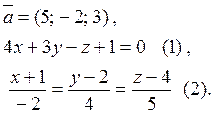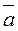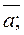# Тестові завдання з аналітичної геометрії (англійською мовою)

### Содержание работы

Individual homework№ 3

І. А(N-3; 1), В(1; N-6;), С(N-10; 2).

1. Find the equations:

1.  the straight line АВ;

2.  the height of triangle АВС, which passes through the vertex В;

3.  the straight line, which passes through the vertex С and parallel to the straight line АВ;

4.  the median of DАВС, which passes through the vertex С;

5.  the median perpendicular to the side АС.

2. Solve the tasks:

1)  Find the length of the height of DАВС, which passes through the vertex С.

2)  Find the projection of the point А on the straight line ВС.

3) Find the angle between the line АВ and line  2x-3y=0.

ІІ.: М1(N; 3; -1), М2(2; N; 4), М3(5; -2; 3),1. Find the equations of the plane:

1) which passes through the point М1 and perpendicular to the vector;

2) which passes through the point М1 and parallel to the plane (1);

3) which passes through the points М123 ;

4) which passes through the point М3 and perpendicular to the straight line  (2).

2. Find the equations of the straight line:

1) which passes through the point М2 and parallel to the vector2) which passes through the point М3 and parallel to the straight line  (2);

3) which passes through the points М1 and М2;

4) which passes through the point М3 and perpendicular to the plane (1).

3. Solve the tasks:

1) Find the angle between the plane (1) and plane from 1.1).

2) Find the angle between the straight line (2) and the plane from 1.1).

3) Find the distance from the point  М1 to the plane (1).

4) Fine the point of the intersection of the straight line (2) and the plane from 1.1).

### Информация о работе

Тип:
Тестовые вопросы и задания
Размер файла:
34 Kb
Скачали:
0